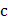# Ifis one of the formthen positive value ofis

## Question ID - 153447 :- Ifis one of the formthen positive value ofis

3537

 (5) Hereis a repeated root of the equationhenceis also a root of the equationi.e.,Ororhas the rootonce which can be either, or 1 IfthengivesorIfthengivesis non-differentiable at. Then the value ofis (whererepresents greatest integer function)